# Algebraic operator

A linear operator satisfying a polynomial identity with scalar coefficients.

Letbe a linear space over a field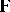. Let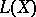be the set of all linear operators with domains and ranges inand let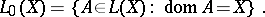Denote by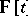the algebra of of all polynomials in the variableand with coefficients in. A linear operatoris said to be algebraic if there exists a non-zero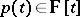such that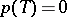(cf. [a2]). Note that I. Kaplansky in [a1] considered rings with a polynomial identity (cf. also PI-algebra).

Usually, in applications it is assumed that the fieldis of characteristic zero and algebraically closed (cf. also Algebraically closed field; Characteristic of a field).

An algebraic operator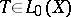is said be of orderiffor asuch that the degree ofisandfor any polynomial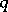of degree less than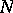. If this is the case, then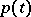is also said to be the characteristic polynomial ofand its roots are called the characteristic roots of. Here and in the sequel it is assumed that the polynomialis normalized or monic, i.e. the coefficient at the highest power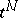is equal to. Algebraic operators with characteristic polynomial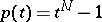(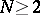) are said to be involutions of order. Their characteristic (single) roots areth roots of unity. Involutions of orderare also briefly called involutions.

An operatoris algebraic of orderif and only if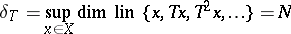(cf. [a2]).

In order to give another characterization of algebraic operators, more useful in applications, write for any-times differentiable functionin an interval containing different points,Let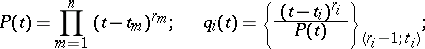Then, by the Hermite interpolation formula with multiple knots, one obtains a partition of unity:This representation is unique, provided thatand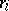are fixed. If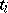are single knots (i.e.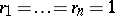), then the Hermite formula yields the Lagrange interpolation formula andLet. Then the following conditions are equivalent (cf. [a4]):

i)is an algebraic operator with characteristic polynomial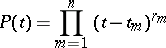of order;

ii) the operators(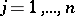) are disjoint projectors giving a partition of unity: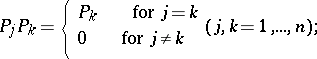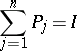(where byis denotes the identity operator in) and such that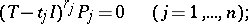iii) the spaceis the direct sum of the principal spaces of the operatorcorresponding to the eigenvaluesof multiplicities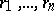respectively (cf. also Eigen value), i.e.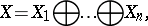where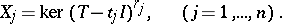If the roots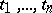are single, then iii) can be formulated as follows:

iv) the space X is the direct sum of eigenspaces of the operatorcorresponding to the eigenvalues:, where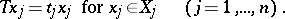An immediate consequence of these conditions is the classical Cayley–Hamilton theorem: If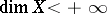, then every operatoris algebraic and its characteristic polynomial is a divisor of the polynomial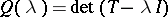(where to each operatorthere corresponds a unique square matrix denoted by the same letter). In that case the characteristic polynomial is said to be minimal.

Ifis algebraic, thenfor every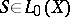and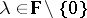(cf. Locally algebraic operator).

In the same manner one can define algebraic elements in an algebra. In that case, the elementsare idempotents giving a partition of unity. If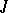is a two-sided ideal in an algebra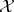and the coset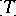corresponding to an elementis an algebraic element in the quotient algebra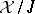(cf. also Rings and algebras), thenis said to be an almost algebraic element. By definition, ifis the characteristic polynomial of, then.

In appropriate spaces, several integral transforms are algebraic; for instance, the Hilbert transform, the Fourier transform, and the Cauchy singular integral on a closed curve (cf. also Cauchy integral). The cotangent Hilbert transform is an almost algebraic operator. The usual translation by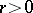is an algebraic operator in the spaces of periodic, exponential-periodic and polynomial-exponential-periodic functions (i.e. linear combinations of products of polynomial, periodic and exponential functions, respectively), provided that the period of the functions under consideration is commensurable with. The so-called Carleman shift of the argument of a function is also an algebraic operator (cf. [a5], [a7]).

Properties of algebraic and almost algebraic operators and elements are very useful in solving several problems involving these operators, in particular those involving the operators listed above, and in several kinds of integral and ordinary and partial differential equations with transformed argument. A particular advantage is that the equivalence of the conditions i)–ii) permits one to reduce a problem under consideration to a problem without any transformation of argument and, eventually, to determine solutions in closed form (cf. [a5], [a6], [a7]).

Other generalizations (for instance, operators satisfying a polynomial identity with non-scalar coefficients) and their applications are examined in [a3].